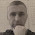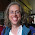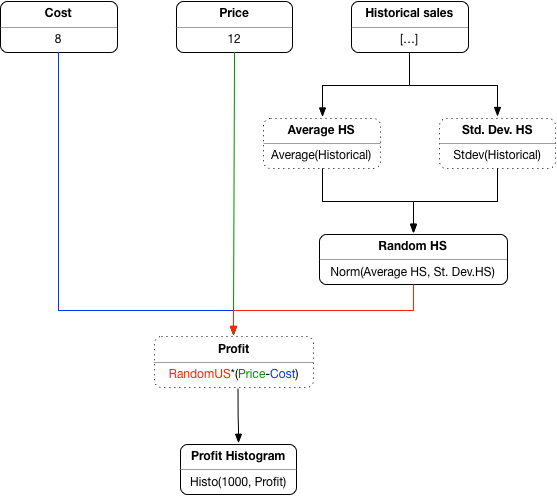## Monday, 30 July 2018

Monads have exerted a curious fascination on programmers who turn to Haskell. The incomprehension of the newbies is matched in intensity only by the smugness of the experts. The official monadic doctrine, as espoused by the Haskell Wiki, is that

Monads in Haskell can be thought of as composable computation descriptions. The essence of monad is thus separation of composition timeline from the composed computation's execution timeline, as well as the ability of computation to implicitly carry extra data, as pertaining to the computation itself, in addition to its one (hence the name) output, that it will produce when run (or queried, or called upon). This lends monads to supplementing pure calculations with features like I/O, common environment, updatable state, etc.
Some of the words above, especially the meandering second sentence, seem to be written to baffle rather than inform. (It reminds me of what Orwell criticises here.) The lay interpretation is that monads are mathemagical constructs that allow you to add "computations" (also sometimes called "effects") to a "pure" programming language. This lay interpretation is largely wrong and the Haskell monadic dogma is rather overblown. In this post I will point out its inaccuracies then explain the real reason why monads are required in Haskell to handle interactive computations. In the process maybe we will understand monads a bit better. Or, at least, maybe we will manage to demystify them.

Monads were introduced by Moggi in his seminal paper Notions of Computation and Monads as a mathematical (categorical) framework for something that he calls computations. The paper is one of the most influential papers in semantics of programming languages. It is mathematically brilliant, well written, and it changed the field. Here are Moggi's original examples of computations:
• partiality, i.e. non-terminating computations
• nondeterminism, i.e. computations that may produce different values
• side-effects, such as reading and writing from state
• exceptions, i.e. computations that may terminate abruptly
• continuations, a powerful mechanism for manipulating flow of control
• interactive input and output
Note that partiality and exceptions are features of what passes for "pure" Haskell. Partial behaviour is what happens when you run a recursive function which never stops, and exceptional behaviour when you try to take the head of an empty list. Such programs are standard Haskell programs which do not produce compiler errors or warnings. And, of course, they are computations in the sense that they do not produce values, as 1+7 or head do.

So it turns out you don't need monads in the type system to have computations. Monads, as a mathematical concept, can be always used as a part of the semantic model, but whether they transpire into the type system of the object language is a matter of design choice. Indeed, one of the most interesting contributions of Moggi's original paper is showing how a monadic meta-language can interpret a language with computations, and how different evaluation strategies require different translations of the (simple) types of the object language into monadic meta-language types.

And of course many functional programming languages have computations but do not require monads. The ML family of languages  (OCaml, SML) have a rich set of computational features which are not reflected in the type system, and so do the grandfather of modern functional languages, ALGOL 60, or more recent  inventions such as Scala.

So that is a bit strange, isn't it? Some computations (partiality, exceptions) do not require language-level monads while others (side-effects, input-output) do. What is the reason for this disparity in Haskell? Is it just a matter of taste? Why do some (most) functional languages make all computations irrelevant to the typing discipline, whereas Haskell makes them relevant -- but only some?

My answer has two parts. In the first part we will see why some Haskell computations need monads when other languages don't. In the second part we will understand why Haskell doesn't need to track all computations monadically.

Most functional languages (ML-family, Scala, etc.) have an "eager" (call-by-value)  evaluation strategy in which a function application f m is evaluated by first bringing the function f and the argument m to a "value" form and then performing the function call. This implies an "evaluation order" so that for example f is evaluated first, then m. The order is language-specific. In this case, if f and m are computations that interact with the world in some way (read or write from state or files) then all the interactions of f will happen before those of m. The schedule of interactions is predictable from the syntactic form of the application f m.  So consider for example assignment, where x:=m can be thought of simply as the infix syntactic form of assign x m. If an assignment is used then the arguments will be evaluated to values (e.g. x to some memory location, m to some integer value) in the language-specific order. The assignment (a computation) will be executed last. If x and m are just variables then we know that variables are bound to values, so they will produce no interactions. The only interaction produced by the term is the assignment itself.

ALGOL 60 works differently, being a "call-by-name" (non-strict) language. This means that in an application f m the argument f is evaluated but m is not, and it's passed as a thunk (Think something like f (fun ()->m) in an ML language.). If f and m are computations then no interactions happen in the application f m. To find the interaction you have to look deeper into the code and find the terms that force evaluations. To repeat the example above, assignment is one of those context when an evaluation must be forced, in order to obtain the location and the assigned value. So the behaviour is similar to the above. However, if x and m are just variables, the behaviour is different because variables are bound to thunks rather than values. And these thunks will be executed. So in a term such as x:=m+m the interactions of x are executed once and those of m twice. The assignment itself, another interaction, will be performed at the end. The schedule of interactions in ALGOL 60 takes more getting used to than in eager languages, because some contexts force evaluation (e.g. arithmetic-logic operators, assignments, etc.) and some don't.  Irregular behaviour is usually conducive to programmer mistakes, so one could speculate that this is a reason why call-by-name didn't catch on. (Note that Haskell also enjoys this sometimes baffling mixing of forcing ("eager") and non-forcing ("lazy") behaviour in pattern matching. To have a principled mix of function call strategies my colleague Paul Levy has proposed a calculus called call-by-push-value.)

However, in both cases of call-by-value and call-by-name the schedule of interactions is syntactically identifiable by a local code inspection. What would happen if we added interactive computations to Haskell in the same way? Does an evaluation f m trigger any interactions? No, because the calling mechanism is lazy. What about an assignment x:=m? Here is the crazy part: we wouldn't know just by looking at the assignment statement itself! In a lazy language the terms bound to x and m are evaluated once, then their values are memoized. And, by necessity, the interactions which are embedded in the computation are carried out during the evaluation. Once we obtain the value the interactions are lost. So in order to know whether an occurrence of m or x produces any interactions you have to inspect the entirety of the code and figure out if it had been evaluated anywhere earlier or not. This is a difficult problem.

This is where monads rescue Haskell. The key relevant construct of the monad is what Moggi called the extension of a function f to a function f*, i.e. the way functions are to be "lifted" to be interpreted in the monadic setting. It is defined as f* c = let x <= c in f x. This helps because it forces the evaluation of argument c in a predictable way. That is all that the monads really need to do in Haskell: turn a language with an unpredictable evaluation strategy into a language with a predictable, sequential evaluation strategy. (The order of evaluation becomes even more apparent when the Haskell "do" notation is used.) This forcing action is emphasised in Moggi's paper:
Remark 2.6 The let-constructor plays a fundamental role: operationally it corresponds to sequential evaluation of programs [...]
The way this is practically achieved in Haskell is to allow the combination of computations only through special "binding operators" (such as >>= and >>) whereas in languages with more predictable evaluation strategies any term may contain sub-terms which contribute computational interactions. Here is a typical implementation (also from the Haskell wiki), where you can see how action1 is explicitly scheduled to be performed before action2:

(>>=) :: IO a -> (a -> IO b) -> IO b
(action1 >>= action2) world0 =
let (a, world1) = action1 world0
(b, world2) = action2 a world1
in (b, world2)

(An aside about the example: The let (x,y)=z pattern matching in Haskell is lazy, so the evaluation is not forced by the pattern matching, as one might reasonably expect, but presumably by the actions. It looks like some monads defined using this recipe might not actually even force sequential evaluation at all, so they may not be monads, technically speaking. Perhaps bang patterns should be used instead?)

There is a bit more to the monad story though. Monads can be merely used as a functional design pattern which has nothing to do with effects -- for example the list monad or the option monad. These monads can be used in any functional languages if so desired. This is why conflating monads and effects is confusing.

Let us revisit our running example of an assignment operation, which you would write as x:=m in most languages. In Haskell you would need to write something like do v <- x; e <-m; assign v e which first force terms x then m to produce values v then and exercise their interactions in the process, then produce the actual assignment via assign. (The actual syntax may end up being more complicated due to difficulties of mixing computations via mixing monads.) At any rate, whether such elaborations are to be considered an improvement is certainly a matter of personal taste.

#### Why are partiality and exceptions invisible to the Haskell type system?

The answer is a pragmatic one. Partial and exceptional computations produce at most one answer, whereas interactive (or non-deterministic) computations may produce multiple answers. Reading variable x for example produces different values at different times. Or, to put it otherwise, m = m evaluates to true, if it evaluates at all, in the presence of partiality and exceptions, whereas m = m may evaluate to false in the presence of interactive computations. The fact that m = m is true-or-bust is what is called "referential transparency" in Haskell, and is a powerful property which allows terms to be treated as if they are values and not computations, thus enabling a wide range of compiler optimisations. (See a related observation in an earlier post.)

I say "as if" because they are not values but computations -- but if the computation is manifested then the whole program either terminates abruptly (exceptions) or never (partiality), so the wrong answer is never produced. This is the idea of "partial correctness", by the way. So these computations are in some sense "benign" and don't need to be tracked.  Because monads are used in association with certain kinds of interactive computations, and not for benign computations, in Haskell they can serve as a simple kind of "effect type system", which is I suppose a genuine but incidental bonus.

By contrast, languages such as Agda take purity very seriously, only allowing computations which are guaranteed to produce one (and only one) value. These languages require the static checking both of exhaustive pattern matching and of termination, which makes them harder to program in. But they are "like maths", or rather "like logic", in a formal sense (see the Curry-Howard correspondence) and can be used dually as programming languages or as proof assistants.

To conclude, if I had control of the Haskell wiki, this is how I would start the page on Monads:
Monads in Haskell are used as a mechanism for scheduling evaluation, thus turning a language with a hard-to-predict evaluation strategy into a language with predictable, sequential, interactions. This makes it possible to add interactive computations such as state and input-output to the language, noting that benign (non-interactive) computations are already a part of the language, transparent to the type system.

1.Front page of hacker news!
https://news.ycombinator.com/item?id=17645277

2.3.Awesome. Priceless insight into the role monads play with Haskell's default lazy evaluation.

4.An expert Haskeller pointed out to me that this point has been made by Simon Peyton Jones in "Wearing the hair shirt"! However my elaboration might help understand what he meant.

5.Simon Marlow pointed this paper to me: "A History of Haskell: Being Lazy With Class" written by Hudak, Hughes, Peyton-Jones, and Wadler. The relevant sections are 3.2 and 7. I don't think there is technical disagreement between what I am saying and what their paper says on the matter, but maybe the emphasis is a bit different. I hugely recommend the paper, although it has some slight inaccuracies.

For example the commend below (from Sec. 3.2) is not technically true, since function f may have a pattern-matching failure or it may diverge. A "partial function" would be more accurate:

For example, if a function f has type Int -> Int you can be sure that f will
not read or write any mutable variables, nor will it perform any
input/output. In short, f really is a function in the mathematical
sense: every call (f 3) will return the same value.

6.Hello. I think it is good to point out that even with Monads Haskell evaluations is not *so* strict. For instance, look at this program:

import System.IO
main :: IO ()
main = do
contents <- hGetContents handle
hClose handle
putStr contents

Assuming the file "foo.txt" is readable, the output of this program is:

Main: foo.txt: hGetContents: illegal operation (delayed read on closed handle)

What happened? Haskell left line 5 (contents <- hGetContents handle) to be evaluated AFTER line 6 (hClose handle) because the variable contents is only required in line 7 (putStr contents).

1.Indeed! Sequencing of effects can be lazy but this is not a good thing. I think I said that in the post but not clearly enough. (I recommended the use of bang pattern matching to force evaluation.)

7.How does tardis and reverse state monad fit in this interpretation?

8.Nice one Dan. I can make the observation technical. Partiality, nondeterminism and exceptions (the last one with some trickiness) are *commutative* monads. So, we can get away with a wink. But with state and I/O, we really do need monads.

1.Yes, that's true. But not all commutative monads are type transparent though. Why?

9.Nice post.
I think it is also worth noting that what first seems to be a disadvantage: Haskell's lazy evaluation mechanism doesn't allow us to integrate effects into the core language turns out to be an advantage. Monads enable a sort of effect polymorphism: we can easily integrate new effects into Haskell. Examples are quantum computations (the QIO monad) and STM (transactional memory).

1.Agreed and I said that in the post although not emphatically. However if a type effect system is what you want, there might be better ways, which achieve more precision than monads.

### Making OCaml more like Excel

Excel, spreadsheet programming in general, is broadly considered a very accessible style of programming. Could we make functional programmin...